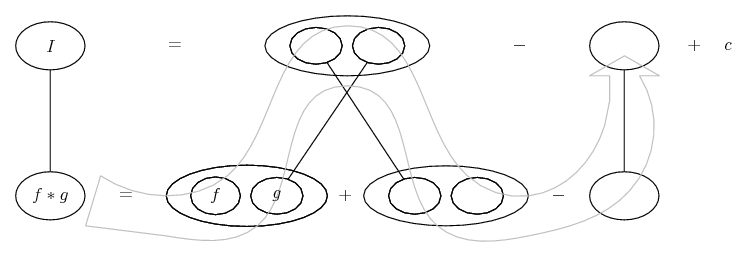# Thread: frustrating indefinite integral

1. ## frustrating indefinite integral

I need to integrate $\displaystyle x^9\sqrt{x^5-3}$
I just cant seem to get the answer my calculator gives me and I could really use some help on this one!

2. ## Re: frustrating indefinite integral

use the substitution - $\displaystyle t=x^5$ you'll end up getting $\displaystyle \int \frac{t\sqrt{t-3}}{5}dt$

think you can carry on from here.

3. ## Re: frustrating indefinite integralOriginally Posted by BAdhiuse the substitution - $\displaystyle t=x^5$ you'll end up getting $\displaystyle \int \frac{t\sqrt{t-3}}{5}dt$

think you can carry on from here.
With another substitution $\displaystyle \displaystyle u = t - 3$4. ## Re: frustrating indefinite integral

why not just use one substitution $\displaystyle s = x^5 - 3$?

5. ## Re: frustrating indefinite integralOriginally Posted by NeedDirectionI need to integrate $\displaystyle x^9\sqrt{x^5-3}$
I just cant seem to get the answer my calculator gives me and I could really use some help on this one!
Integration by parts with:

$\displaystyle u=x^5$

and

$\displaystyle dv=x^4 \sqrt{x^5-3}$

CB

6. ## Re: frustrating indefinite integral

Thanks guys, I finally got it worked out! I couldn't figure out the last way of doing it with integration by parts but oh well, whatever works!

7. ## Re: frustrating indefinite integralOriginally Posted by NeedDirectionoh well, whatever works!
Absolutely. But, the parts way is too neat not to have been the point of the puzzle. So, just in case a picture helps...... where (key in spoiler) ...

Spoiler:... is the product rule, straight continuous lines differentiating downwards with respect to x.Then, for the other pic, we have also...... the chain rule. Straight continuous lines differentiate downwards (integrate up) with respect to the main variable (in this case x), and the straight dashed line similarly but with respect to the dashed balloon expression (the inner function of the composite which is subject to the chain rule).

(Wrapped inside the legs uncrossed version of the product rule.)

Zooming in on the chain rule (and, to that end, un-crossing the legs of the product rule), and following through...Full size

__________________________________________________ __________

Don't integrate - balloontegrate!

Balloon Calculus; standard integrals, derivatives and methods

Balloon Calculus Drawing with LaTeX and Asymptote!

8. ## Re: frustrating indefinite integral

the integration by parts illustrates why the substitutions work: splitting x^9 into x^5 and x^4 gives you something outside the radical, which is the derivative (well, almost) of the beastie inside the radical. and this is good, because as any conservative will tell you, radicals are evil, and need to be monitored closely.

#### Search Tags

frustrating, indefinite, integral